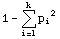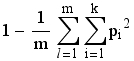Lecture 4.  Population Genetics II

Heterozygosity, HExp (or gene diversity, D)

Heterozygosity is of major interest to students of genetic variation in natural populations. It is often one of the first "parameters" that one presents in a data set. It can tell us a great deal about the structure and even history of a population. Just for example, very low heterozygosities for allozyme loci in cheetahs and black-footed ferrets indicate severe effects of small population sizes (population bottlenecks or metapopulation dynamics that severely reduced the level of genetic variation relative to that expected or found in comparable mammals).  High heterozygosity means lots of genetic variability.  Low heterozygosity means little genetic variability.  Often, we will compare the observed level of heterozygosity to what we expect under Hardy-Weinberg equilibrium (HWE).  If the observed heterozygosity is lower than expected, we seek to attribute the discrepancy to forces such as inbreeding.  If heterozygosity is higher than expected, we might suspect an isolate-breaking effect (the mixing of two previously isolated populations).

Several measures of heterozygosity exist. The value of these measures will range from zero (no heterozygosity) to nearly 1.0 (for a system with a large number of equally frequent alleles).  We will focus primarily on expected heterozygosity (HE, or gene diversity, D, as Bruce Weir prefers to call it). The simplest way to calculate it for a single locus is as:Eqn 4.1
where pi is the frequency of the ith of k alleles. [Note that p1, p2, p3 etc. may correspond to what you would normally think of as p, q, r, s etc.]. If we want the gene diversity over several loci, we need double summation and subscripting as follows:Eqn 4.2
where the first summation is for the lth ("ellth") of m loci. [Note that we average over the m loci via the 1/m term].  The second summation is as in Eqn 4.1.

Why does it work to take the sum of the squared gene frequencies and subtract that from one? Lets think back to basic Hardy-Weinberg:

p2 + 2 pq + q2 = 1                                                                                             Eqn 4.3
where the heterozygosity is given by 2pq. The rest of the expression (p2 + q2) is the homozygosity. If we want the heterozygosity, we just subtract that from the total. With just two alleles it isn't as efficient to calculate the heterozygosity by the "one minus the homozygosity route". Consider the case, though, of a locus with 6 alleles. It has 21 possible genotypes -- 6 kinds of homozygotes and 15 kinds of heterozygotes.  Writing it out, 6 + 5 + 4 + 3 + 2 + 1 = 21 = [6*(6+1)]/2 -- this is the formula for combinations of six things taken two at a time, order unimportant -- [n(n+1)] / 2. The more alleles, the simpler it becomes simply to square the gene frequencies and sum then, compared to enumerating all possible heterozygotes and calculating the (possibly very many) different heterozygote frequencies. We trade a little inefficiency on two-allele systems for much greater efficiency with multi-allele systems.

What does heterozygosity tell us, and what patterns emerge as we go to multi-allelic systems? Lets take an example. Say p = q = 0.5. The heterozygosity for a two-allele system is described by a concave down parabola that starts at zero (when p = 0) goes to a maximum at p = 0.5 and goes back to zero when p = 1. In fact, for any multi-allelic system, heterozygosity is greatest when

p1 = p2 = p3 = .pk                                                                     Eqn 4.4
that is, when the allele frequencies are equal. The maximum heterozygosity for a 10-allele system comes when each allele has a frequency of 0.1 -- D or HE then equals 0.9.  Later, we will see that the simplest way to view FST (a measure of the differentiation of subpopulations) will be as a function of the difference between the Observed heterozygosity, Ho, and the Expected heterozygosity, HE,  that we have just derived.

Individuals-eye view of heterozygosity

Here is a way that I like to think of heterozygosity (HE or D). It is the (expected) probability that an individual will be heterozygous at a given locus (or over the assayed loci for a multi-locus system). For many human microsatellite loci, for example, HE is often > 0.85, meaning that you have a > 85% chance of being a heterozygote.

Now that you have a way to calculate gene diversity/expected heterozygosity, you are ready to calculate F-statistics by the method of:

FIS = (HS - HI) / HS                                                                        Eqns 4.5

FST = (HT - HS) / HT

FIT = (HT - HI) / HT

As shown in the worked F-statistic web page demo.

If you run some data through Eqns 4.5 and an analysis program you may ask:

"Why is the FST I calculate with FSTAT (or some other software)

different from the one I calculate using Eqns 4.5?"
Answer: because the analysis programs use more complex algorithms that take into account such factors as how individuals disperse (island model vs. stepping-stone model vs. lattice model), the mutation process (infinite alleles model vs. stepwise mutation model) and various bias adjusters (e.g., taking into account the sample size of the subpopulations sampled).